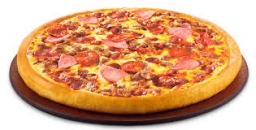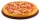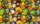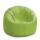# Pizza fractions

Ann ate a third of a pizza and then another quater. Total part of pizza eaten by Ann and how much pizza is left?

e =  0.4167
l =  0.5833

### Step-by-step explanation:

$e=1-1\mathrm{/}3-1\mathrm{/}4=\frac{5}{12}=0.4167$
$l=1-e=1-0.4167=\frac{7}{12}=0.5833$Did you find an error or inaccuracy? Feel free to write us. Thank you!Tips to related online calculators
Need help to calculate sum, simplify or multiply fractions? Try our fraction calculator.

## Related math problems and questions:

• Pizza 4Marcus ate half pizza on monday night. He than ate one third of the remaining pizza on Tuesday. Which of the following expressions show how much pizza marcus ate in total?
• Bitoo and ReenaBitoo ate 3/5 part of an apple and the remaining part was eaten by his sister Reena. How much part of an apple did Renna eat? Who had the larger share? By how much?
• PizzaThree siblings ordered one pizza. Miška ate a quarter of the whole pizza. Lenka ate a third of the rest and Patrik ate half of what Lenka had left. They had the rest packed up. How much of the pizza did they pack? Write the result as a fraction.
• Two pizzasJacobs mom bought two whole pizzas. He ate 2/10 of the pizza and his dad ate 1 1/5. How much is left.
• Roma ateRoma ate 2/5 of a cake while Somya ate 3/7 of the same cake. Who ate more and by how much?
• PizzasBilly ate 1 1/4 pizzas and John ate 1 2/3 pizzas. How much more pizza did John eat than Billy?
• Grandmother and grandfatherGrandmother baked cakes. Grandfather ate half, then quarter of the rest ate Peter and Paul ate half of rest. For parents left 6 cakes. How many cakes maked the grandmother?
• CupcakesIn a bowl was some cupcakes. Janka ate one third and Danka ate one quarter of cupcakes. a) How many of cookies ate together? b) How many cookies remain in a bowl? Write the results as a decimal number and in notepad also as a fraction.
• IssacIssac eats 1/6 of the pizza. Maya then eats 3/5 of the remaining pizza. What fraction of the original pizza is left?To three-eighths of one third, we add five quarters of one half and multiply the sum by four. How much will we get?
• CerealsAri and Joey share a 30-ounce box of cereal. By the end of the week, Ari has eaten 3/10 of the box, and Joey has eaten 3/5 of the box of cereal. How many ounces are left in the box?
• Unknown numberI think the number. I'll reduce it to its one-third. The result is then increased by one-third, and I get the number 12.
• CandiesThere are 150 candies in the bowl. Ivan took 7 thirtieths, Charles 6 fifteenths, and Thomas 3 tenths. How many sweets are left in the bowl?
• SavingsEva borrowed 1/3 of her savings to her brother, 1/2 of savings spent in the store and 7 euros left. How much did she save?
• The sum 12The sum of 3 mixed numbers is 20 13/15. two of the numbers are 6 1/3 and 7 5/6. what is the third number?
• Farmer 5Farmer Joe ordered 3 bags of soil last month. Each bag weighed 4 ⅖ kilograms. He used the first bag in a week. At the end of this month, there were 2 ¾ kilograms of soil left in the second bag and ⅞ kilograms of soil left in the third bag. How much soil w
• Slices of pizzaMaria ate 1/4 of a pizza. If there were 20 slices of pizza, how many slices did Maria eat?